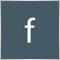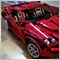# order management problem218

hi i created this simple concept of my expert so i can show you whats the problem

expert has to run in diffrent charts of the same currency pair so i have to apply magic number to each order and the basic concept of it is that i have to open the next order based on the results of the last one

for  example a sell if the previous orders hit stoploss a buy if it hits tp

the expert works fine until it goes up ...for example the first order  which is a buy hits tp and it has to open a sell well it opens a sell and it opens a buy which was the previous order at the same time

instead of one order it opens twp orders the order that it should open and again the previous one

from my tests the problem is magic number if i dont apply magic number the problem is solved but i have to apply it.. so im kinda stuck

here is my code

```double pips;
extern int magic_n=88229;
extern int magic_n2=29522;

//+------------------------------------------------------------------+
//| Expert initialization function                                   |
//+------------------------------------------------------------------+
int OnInit()
{
//---

//APPLYING PIPS
double ticksize=MarketInfo(Symbol(),MODE_TICKSIZE);
if(ticksize==0.00001||ticksize==0.001)
pips=ticksize*10;
else pips=ticksize;

//---
return(INIT_SUCCEEDED);
}
//+------------------------------------------------------------------+
//| Expert deinitialization function                                 |
//+------------------------------------------------------------------+
void OnDeinit(const int reason)
{
//---

}
//+------------------------------------------------------------------+
//| Expert tick function                                             |
//+------------------------------------------------------------------+
void OnTick()
{
//---

for(int i=OrdersHistoryTotal()-1;i>=0;i--)
{
if(OrderSelect(i,SELECT_BY_POS,MODE_HISTORY))
if(OrderMagicNumber()==magic_n)
{
if(OrderClosePrice()==OrderStopLoss())
{
if(open_pairr(Symbol(),magic_n2)<1)
{
}
}
else if(OrderClosePrice()==OrderTakeProfit())
{
if(open_pairr(Symbol(),magic_n2)<1)
{
entry(Symbol(),OP_SELL,0.03,0,5,35,35,NULL,magic_n2);
}
}
}

}
//THE FIRST ORDER
if(open_pairr(Symbol(),magic_n)<1)
{
entry(Symbol(),OP_SELL,0.01,0,5,35,35,NULL,magic_n);

}
}
//+-----------------------------------------------------------------+
//THE FUNCTION FOR APPLYING MAGIC NUMBER AND SYMBOL TO THE TRADES
int open_pairr(string pair,int magic)
{
int total=0;
for(int i=OrdersTotal()-1;i>=0;i--)
{
{
if(OrderSymbol()==pair&&OrderMagicNumber()==magic)
{
total++;
}
}
}
}
int send_order(string ins,int cmd,double volume,int distance,int slippage,int sl,int tp,string comment=NULL,int magic=0,int expire=0,int a_clr=clrNONE,bool market=false)
{
double price=0;
double price_sl = 0;
double price_tp = 0;
double point=MarketInfo(ins,MODE_POINT);
datetime expiry= 0;
int order_type = -1;
RefreshRates();
{
if(!market)
{
if(sl>0) price_sl = price-sl*pips;
if(tp>0) price_tp = price+tp*pips;
}
}
else if(cmd==OP_SELL)
{
if(distance>0) order_type=OP_SELLLIMIT;
else if(distance<0) order_type=OP_SELLSTOP;
else order_type=OP_SELL;
if(order_type==OP_SELL) distance=0;
price=MarketInfo(ins,MODE_BID)+distance*pips;
if(!market)
{
if(sl>0) price_sl = price+sl*pips;
if(tp>0) price_tp = price-tp*pips;
}
}
if(order_type<0) return 0;
else  if(order_type==0 || order_type==1) expiry=0;
else if(expire>0)
expiry=(datetime)MarketInfo(ins,MODE_TIME)+expire;
if(market)
{
int ticket=OrderSend(ins,order_type,volume,price,slippage,0,0,comment,magic,expiry,a_clr);
if(ticket>0)
{
if(OrderSelect(ticket,SELECT_BY_TICKET))
{
{
if(sl>0) price_sl = OrderOpenPrice()-sl*pips;
if(tp>0) price_tp = OrderOpenPrice()+tp*pips;
}
else if(cmd==OP_SELL)
{
if(sl>0) price_sl = OrderOpenPrice()+sl*pips;
if(tp>0) price_tp = OrderOpenPrice()-tp*pips;
}
bool result=OrderModify(ticket,price,price_sl,price_tp,expiry);
}
}
return ticket;
}
return OrderSend(ins,order_type,volume,price,slippage,price_sl,price_tp,comment,magic,expiry,a_clr);
}
//+------------------------------------------------------------------+
//|                                                                  |
//+------------------------------------------------------------------+
int entry(string ins,int cmd,double volume,int distance,int slippage,int sl,int tp,string comment=NULL,int magic=0,int expire=0,int a_clr=clrNONE,bool market=false,int retries=20,int sleep=500)
{
int ticket=0;
for(int i=0;i<retries;i++)
{
if(IsStopped()) Print("Expert was stopped");
else if(!IsConnected()) Print("No internet connection");
else if(!IsExpertEnabled()) Print("Experts not enabled in trading platform");
else ticket=send_order(ins,cmd,volume,distance,slippage,sl,tp,comment,magic,expire,a_clr,market);
if(ticket>0)
break;
else Print("Error in sending order ("+IntegerToString(GetLastError(),0)+"), retry: "+IntegerToString(i,0)+"/"+IntegerToString(retries));
Sleep(sleep);
}
return ticket;
}
```1219

Farvardin Faili:

hi i created this simple concept of my expert so i can show you whats the problem

expert has to run in diffrent charts of the same currency pair so i have to apply magic number to each order and the basic concept of it is that i have to open the next order based on the results of the last one

for  example a sell if the previous orders hit stoploss a buy if it hits tp

the expert works fine until it goes up ...for example the first order  which is a buy hits tp and it has to open a sell well it opens a sell and it opens a buy which was the previous order at the same time

instead of one order it opens twp orders the order that it should open and again the previous one

from my tests the problem is magic number if i dont apply magic number the problem is solved but i have to apply it.. so im kinda stuck

here is my code

Looks like this check:

```   //THE FIRST ORDER
if(open_pairr(Symbol(),magic_n)<1)
{
entry(Symbol(),OP_SELL,0.01,0,5,35,35,NULL,magic_n);

}
```

will be passed and entry() will execute every time (in fact, every tick) that there is no order with magic_n open. I assume this code is not in the other EA that you run in another chart?

Another problem is when you check OrdersHistoryTotal(), you should also check the orders' time, otherwise, as time goes by, you'll always get a history order that close by TP or SL, all the time. Not posing huge problem at the moment if you're only running two charts, and you're also checking your magic_n and magic_n2, but the logic is incomplete.20250

```if(OrderClosePrice()==OrderStopLoss())
```
1. SL becomes a market order when hit. Slippage could be multiple pips.

2. Doubles are rarely equal. Understand the links in:
The == operand. - MQL4 programming forum